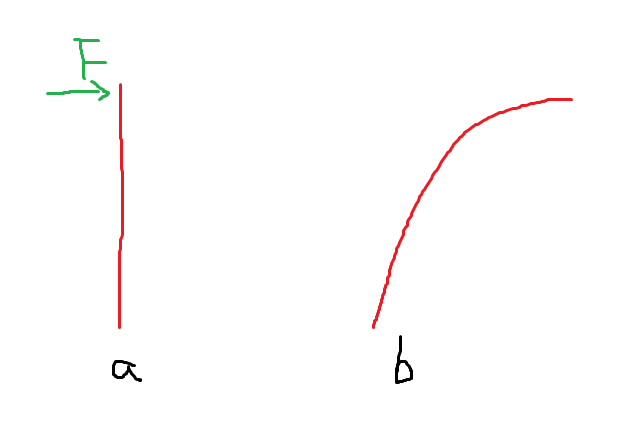# How to apply force to a stick?

I want to use `BulletCylinderShape/BulletConeTwistConstraint` to simulate a stick. Then, I want to move it, and collision happend between it with a static body. For example, the following figure.My question is: how to apply a force in the stick to move it? Or can I just move it forward for a distance? For example, move forward `3m`.

In addition, how to apply force to this stick to make it bend? For example the following figure:In fig a, a force is applied to this stick, and it turn to be bend in fig b. How can I simulate it?

Any suggestion is appreciated~~~

This can be done by these methods by applying them to relative to the center of mass

`applyCentralForce`, `applyCentralImpulse`, `applyImpulse`, `applyForce`, `applyTorque`, `applyTorqueImpulse`

https://docs.panda3d.org/1.10/python/reference/panda3d.bullet.BulletRigidBodyNode#panda3d.bullet.BulletRigidBodyNode.applyCentralForce

1 Like

The input of `applyForce` is `LVector3/LPoint3`. The `LVector3` can represent the direction of force. But, I want to know how to set the magnitude of force?

Does the magnitude of `LVector3` is the magnitude of force? What is the unit? Newton?

In addition, if I want the direction of force is the normal of the stick last point, for example the following picture, should I adjust the force in the `update` function? (`taskMgr.add(update, 'update')`)The update function looks like:

``````def update(task):

### calculation the direction

final_node.clear_forces()
final_node.apply_force(Vec3(force, force, force), Point3(0, 0, segment_length))
dt = globalClock.getDt()
world.doPhysics(dt)
``````direction = Vec3(2, 34, 10)*50